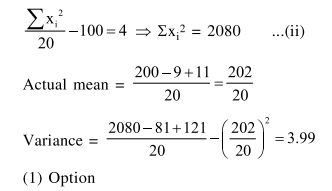Deepak Scored 45->99%ile with Bounce Back Crack Course. You can do it too!

# The mean and variance of 20 observations

Question:

The mean and variance of 20 observations are found to be 10 and 4 , respectively. On rechecking, it was found that an observation 9 was incorrect and the correct observation was 11. Then the correct variance is

1. $3.99$

2. $3.98$

3. $4.02$

4. $4.01$

Correct Option: 1

Solution:

$\frac{\sum x_{i}}{20}=10 \Rightarrow \Sigma x_{i}=200$ .........(i)